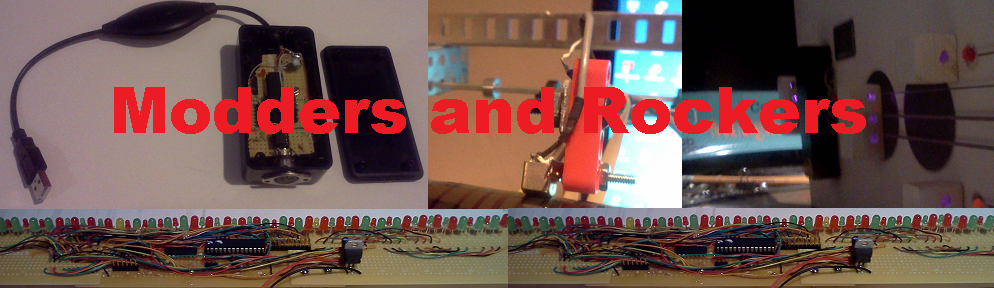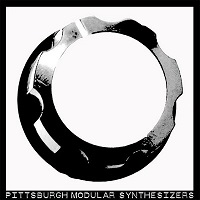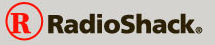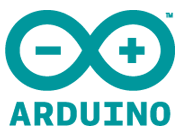# LittleBits Arduino Synth Fun

The Oscillator in the LittleBits Korg Synth Kit has two waveforms. The square waveform and a sawtooth waveform. When using the oscillator as a modulation source to contol the filter cutoff, having only a ramp down sawtooth is a bit limiting. To give more control, the Arduino bit is use to do some simple analog processing.

The code is very simple and gives an example of how the Arduino interface will look when editing, compiling and downloading the code.

 void setup() { } void loop() { int a0; int aout; int a1; a0 = analogRead(0); a1 = analogRead(1); if( a1 > 512){ aout = a0 / 4; a1 = a1 - 512; aout = aout * ( a1 / 2); aout = aout / 256; }else { a1 = 512 - a1; aout = 255 - (a0 / 4); aout = aout * ( a1 / 2); aout = aout / 256; } analogWrite( 5, aout); }